### Home > MC1 > Chapter 8 > Lesson 8.4.2 > Problem8-119

8-119.

Simplify each of the following expressions.

1.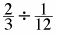2.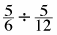3.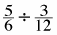4.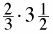5.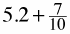6.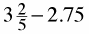Find the common denominators for both fractions. Once you have, divide the numerators to find the answer.

The lowest common multiple of the numbers will also be the common denominator.

$\text{The common denominator is 12, so }\frac{2}{3} = \frac{8}{12}.$

$\text{This means }\frac{2}{3} \div \frac{1}{12} = \frac{8}{12} \div \frac{1}{12}$

Now divide the numerators to solve.

$\frac{8}{1}=8$

2

Change the mixed number to a fraction and then multiply.

$\frac{7}{10} = 0.7,\text{ can you add the decimals?}$

$5.9, \text{ or }5\frac{9}{10}$

$2.75 =\frac{11}{4} \text{ and }3\frac{2}{5} =\frac{17}{5}$

Can you find the common denominator and subtract the fractions?